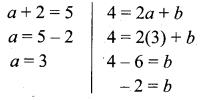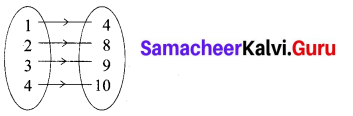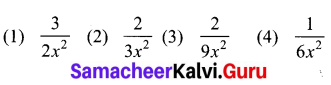## Tamilnadu Samacheer Kalvi 10th Maths Solutions Chapter 1 Relations and Functions Ex 1.6

Question 1.
If n(A × B) = 6 and A = {1, 3} then n(B) is
(1) 1
(2) 2
(3) 3
(4) 6
(3) 3
Hint:
If n(A × B) = 6
A = {1, 1}, n(A) = 2
n(B) = 3

Question 2.
A = {a, b,p}, B = {2, 3}, C = {p, q, r, s)
then n[(A ∪ C) × B] is ………….
(1) 8
(2) 20
(3) 12
(4) 16
(3) 12
Hint: A ∪ C = [a, b, p] ∪ [p, q, r, s]
= [a, b, p, q, r, s]
n (A ∪ C) = 6
n(B) = 2
∴ n [(A ∪ C)] × B] = 6 × 2 = 12Question 3.
If A = {1, 2}, B = {1, 2, 3, 4}, C = {5, 6} and D = {5, 6, 7, 8} then state which of the following statement is true.
(1) (A × C) ⊂ (B × D)
(2) (B × D) ⊂ (A × C)
(3) (A × B) ⊂ (A × D)
(4) (D × A) ⊂ (B × A)
(1) (A × C) ⊂ (B × D)]
Hint:
A = {1, 2}, B = {1, 2, 3, 4},
C = {5, 6}, D ={5, 6, 7, 8}
A × C ={(1,5), (1,6), (2, 5), (2, 6)}
B × D = {(1, 5),(1, 6),(1, 7),(1, 8),(2, 5),(2, 6), (2, 7), (2, 8), (3, 5), (3, 6), (3, 7), (3, 8)}
∴ (A × C) ⊂ B × D it is true

Question 4.
If there are 1024 relations from a set A = {1, 2, 3, 4, 5} to a set B, then the number of elements in B is ………………….
(1) 3
(2) 2
(3) 4
(4) 8
(2) 2
Hint: n(A) = 5
n(A × B) = 10
(consider 1024 as 10)
n(A) × n(B) = 10
5 × n(B) = 10
n(B) = $$\frac { 10 }{ 5 }$$ = 2
n(B) = 2

Question 5.
The range of the relation R = {(x, x2)|x is a prime number less than 13} is
(1) {2, 3, 5, 7}
(2) {2, 3, 5, 7, 11}
(3) {4, 9, 25, 49, 121}
(4) {1, 4, 9, 25, 49, 121}
(3) {4, 9, 25, 49, 121}]
Hint:
R = {(x, x2)/x is a prime number < 13}
The squares of 2, 3, 5, 7, 11 are
{4, 9, 25, 49, 121}Question 6.
If the ordered pairs (a + 2,4) and (5, 2a + 6) are equal then (a, b) is ………
(1) (2, -2)
(2) (5, 1)
(3) (2, 3)
(4) (3, -2)
(4) (3, -2)
Hint:The value of a = 3 and b = -2

Question 7.
Let n(A) = m and n(B) = n then the total number of non-empty relations that can be defined from A to B is
(1) mn
(2) nm
(3) 2mn – 1
(4) 2mn
(4) 2mn
Hint:
n(A) = m, n(B) = n
n(A × B) = 2mn

Question 8.
If {(a, 8),(6, b)} represents an identity function, then the value of a and 6 are respectively
(1) (8,6)
(2) (8,8)
(3) (6,8)
(4) (6,6)
(1) (8,6)
Hint: f = {{a, 8) (6, 6)}. In an identity function each one is the image of it self.
∴ a = 8, b = 6

Question 9.
Let A = {1, 2, 3, 4} and B = {4, 8, 9, 10}. A function f : A → B given by f = {(1, 4),(2, 8),(3, 9),(4, 10)} is a
(1) Many-one function
(2) Identity function
(3) One-to-one function
(4) Into function
(3) One-to one function
Hint:
A = {1, 2, 3, 4), B = {4, 8, 9,10}Question 10.
If f(x) = 2x2 and g (x) = $$\frac{1}{3 x}$$, Then fog is(3) $$\frac{2}{9 x^{2}}$$
Hint:
f(x) = 2x2
g(x) = $$\frac{1}{3 x}$$
fog = f(g(x)) = $$f\left(\frac{1}{3 x}\right)=2\left(\frac{1}{3 x}\right)^{2}$$
= 2 × $$\frac{1}{9 x^{2}}=\frac{2}{9 x^{2}}$$Queston 11.
If f: A → B is a bijective function and if n(B) = 7 , then n(A) is equal to …………..
(1) 7
(2) 49
(3) 1
(4) 14
(1) 7
Hint:
n(B) = 7
Since it is a bijective function, the function is one – one and also it is onto.
n(A) = n(B)
∴ n(A) = 7

Question 12.
Let f and g be two functions given by f = {(0, 1), (2, 0), (3, -4), (4, 2), (5, 7)} g = {(0, 2), (1, 0), (2, 4), (-4, 2), (7, 0)} then the range of fog is
(1) {0, 2, 3, 4, 5}
(2) {-4, 1, 0, 2, 7}
(3) {1, 2, 3, 4, 5}
(4) {0, 1, 2}
(4) {0, 1, 2}
Hint:
gof = g(f(x))
fog = f(g(x))
= {(0, 2),(1, 0),(2, 4),(-4, 2),(7, 0)}
Range of fog = {0, 1, 2}

Question 13.
Let f (x) = $$\sqrt{1+x^{2}}$$ then ………………..
(1) f(xy) = f(x) f(y)
(2) f(xy) > f(x).f(y)
(3) f(xy) < f(x). f(y)
(4) None of these
(3) f(xy) < f(x) . f(y)Question 14.
If g = {(1, 1),(2, 3),(3, 5),(4, 7)} is a function given by g(x) = αx + β then the values of α and β are
(1) (-1, 2)
(2) (2, -1)
(3) (-1, -2)
(4) (1, 2)
(2) (2,-1)
Hint:
g(x) = αx + β
α = 2
β = -1
g(x) = 2x – 1
g(1) = 2(1) – 1 = 1
g(2) = 2(2) – 1 = 3
g(3) = 2(3) – 1 = 5
g(4) = 2(4) – 1 = 7

Question 15.
f(x) = (x + 1)3 – (x – 1)3 represents a function which is …………….
(1) linear
(2) cubic
(3) reciprocal